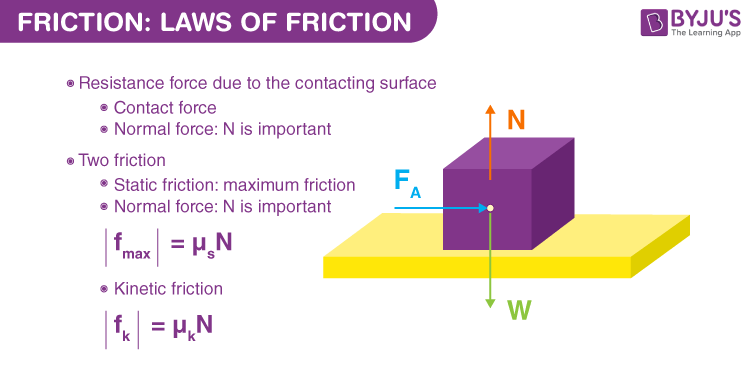# Friction: Laws of Friction

When we throw a ball on the floor, it moves with some velocity. But ideally, no force should be acting in the direction of motion, and according to Newton’s first law, the ball should keep rolling, but this does not happen. Instead, the ball stops after moving a certain distance, so a force must be acting on it. We call this force “friction.” Friction provides traction that is needed to walk without slipping. Friction is helpful in most cases. However, they also offer a great measure of opposition to the motion. In the next section, let us learn about the laws of friction.

## What Are the Laws of Friction?

There are five laws of friction and they are:

• The friction of the moving object is proportional and perpendicular to the normal force.
• The friction experienced by the object is dependent on the nature of the surface it is in contact with.
• Friction is independent of the area of contact as long as there is an area of contact.
• Kinetic friction is independent of velocity.
• The coefficient of static friction is greater than the coefficient of kinetic friction.The laws of friction for two bodies in contact with each other are given as follows:

• If the two bodies in contact with each other are slipping over each other, or having relative motion with respect to each other, the friction that is involved is kinetic friction and is given by:
• $$\begin{array}{l}F_K = µ_k N\end{array}$$
• where,
• fk = kinetic friction
• µk = coefficient of kinetic friction
• N = Normal reaction force
• The direction of this kinetic friction on a body is opposite to the direction of the relative velocity of the body with respect to the other body.

Suppose body A moves over body B in the positive x-direction, then the kinetic frictional force on body A will act in the negative x-direction.

• If the bodies do not have relative motion with respect to each other, the friction between them is static friction and is always less than or equal to the kinetic friction. It is important to remember that the value of static friction is not constant, as the case is with kinetic friction. The value of static friction depends on how much force is applied to the body. Suppose the maximum value of static friction (limiting friction) is 5 N for a body kept on a certain surface. If you apply a force of 2 N on the body, the value of static friction would be 2 N, and the body will not move. The body will start moving when the force crosses the values of 5 N.
• The kinetic frictional force and the static frictional force do not depend on the area of contact as long as the Normal Reaction force is the same.
• The maximum value of static friction is achieved when the body is just on the verge of motion. The friction at this point is also known as limiting friction. Limiting friction is always slightly greater than the kinetic friction and is given by:
• fmax = µs N
• where,
• fmax = limiting friction
• µs = coefficient of static friction
• N = Normal reaction force

## Understand the variable and self-adjusting nature of the static friction with the help of the animation provided in the video below.## Frequently Asked Questions – FAQs

Q1

### How does friction produce heat?

When surfaces in contact move relative to each other, the friction between the two surfaces convert kinetic energy into heat.
Q2

### How is friction useful?

Friction helps us in walking. For example, the friction between the shoes and the floor stops us from slipping. Similarly, the friction between the car and the road prevents the car from sliding.
Q3

### Why is friction a non-conservative force?

Friction is a non-conservative force because the amount of work done by the friction depends on the path.
Q4

### Will friction increase as the speed increases?

No, friction is not affected by the increase in speed.
Q5

### Can friction be zero?

No, it is impossible to have zero friction because every surface will have minor irregularities no matter how lubricated.

## Suggested Videos

#### Introduction to Friction#### Friction on an Inclined PlaneStay tuned to BYJU’S and Fall in Love with Learning!

Test Your Knowledge On Friction Laws Of Friction!